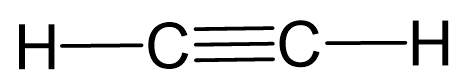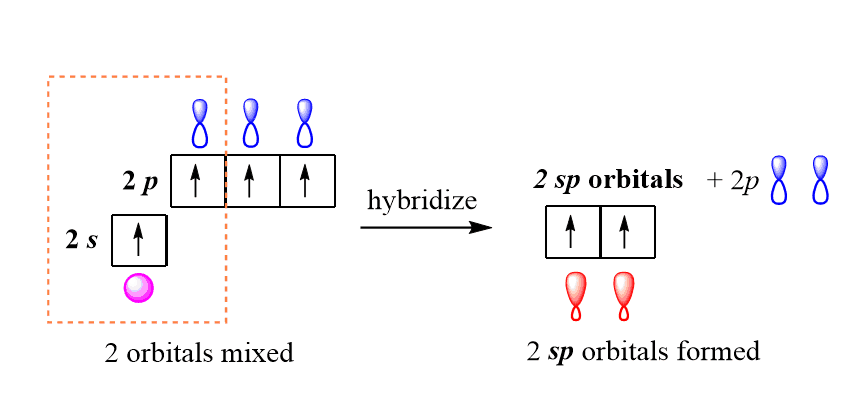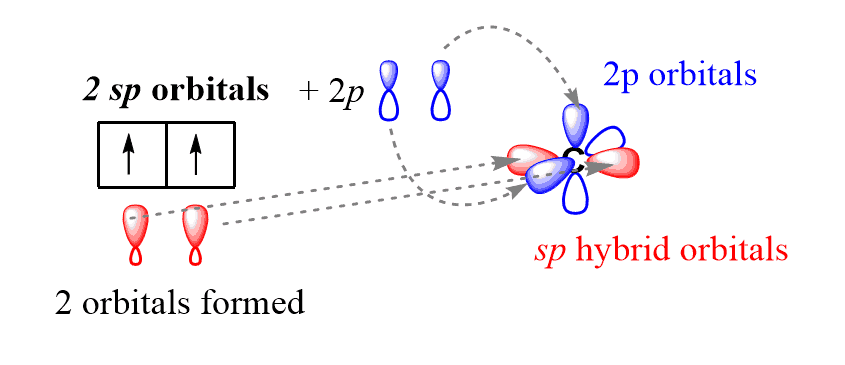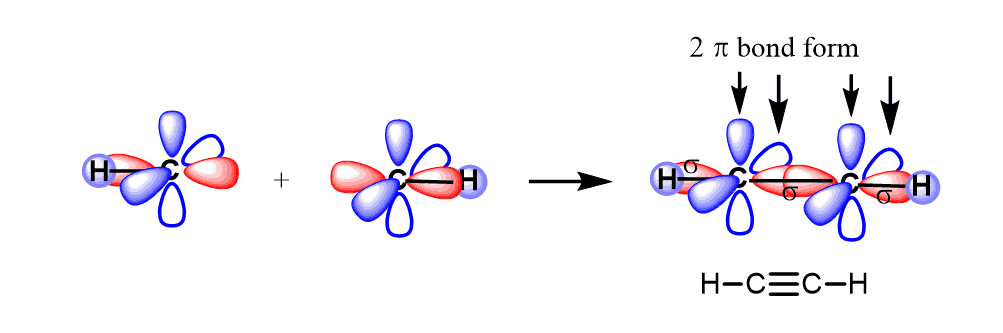\$referrer_host = www.google.com. C2H2 Geometry and Hybridization - Chemistry Steps

## Examples

Because the hydrogens are always in terminal positions, the carbons must be connected, and therefore, we can draw a preliminary skeletal structure to start with:There are 2×4 + 2×1 = 10 valence, and 6 have been used to make 3 covalent bonds. Therefore, the remaining 4 go to the carbon atoms as a lone pair on each:The carbons atoms are lacking octet, so we use the two lone pairs for making 2 additional bonds between them:Even though there is no single central atom, both carbons are linear since their steric number is 2 which makes the molecule linear as well.

Additionally, if you have covered the hybridization theory, you should recognize that both carbons are sp-hybridized and thus the bond angle is 180o which makes the molecule linear.

In sp hybridization, the s orbital of the excited state carbon is mixed with only one out of the three 2p orbitals. It is called sp hybridization because two orbitals (one s and one p) are mixed:The resulting two sp hybrid orbitals are then arranged in a linear geometry (180o) and the two unhybridized 2p orbitals are placed at 90o:Let’s see how this happens in acetylene- C2H2. The two carbon atoms make a sigma bond by overlapping the sp orbitals.One hydrogen bonds to each carbon atom by overlapping its s orbital with the other sp orbital.

The two p orbitals of each carbon overlap to make two π bonds.

The key parameters about the sp hybridization and triple bond:

* All the atoms have linear geometry.

* The angle between atoms is 180o.

* In a triple bond there is one σ (sigma) and two π (pi) bonds.

Check Also

1686019213